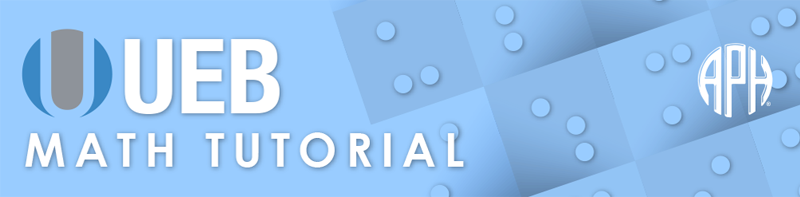Practice Problems -   -  Use 6 Dot Entry   Switch to Nemeth Tutorial

# Lesson 9.3: Circles and Arcs

## Symbols

$\text{arc over previous item}$
⠨⠸⠱

## Review

There are indicators in UEB that apply to the item appearing just before or after the indicator. Braille grouping indicators must be used to enclose an expression that includes more than one item.

The shape indicator has a grade 2 meaning, therefore it must be in grade 1 mode. The shape indicator turns on shape mode and no further grade 1 indicators are needed for the shape. Shape mode is terminated by a space or the shape terminator.

## Explanation

An arc of a circle is a segment of the circumference of the circle. Notation for an arc can be a concave upward or downward curve. The curve notation is shown above the letters of the arc segment in print. The symbol in braille follows the letters of the arc. The arc over previous item consists of three cells, dots four six, dots four five six, and dots one five six. The letters of the arc must be enclosed in braille grouping symbols.

### Example 1

$\stackrel{^}{\mathrm{AB}}=45°$
⠰⠰⠣⠠⠠⠁⠃⠜⠨⠸⠱⠀⠐⠶⠀⠼⠙⠑⠘⠚

### Example 2

$\stackrel{^}{\mathrm{MN}}\phantom{\rule{.3em}{0ex}}\text{is minor.}$
⠰⠰⠣⠠⠠⠍⠝⠜⠨⠸⠱⠀⠊⠎⠀⠍⠔⠕⠗⠲

### Example 3

$m\stackrel{^}{\mathrm{EF}}=40°$
⠰⠰⠍⠣⠠⠠⠑⠋⠜⠨⠸⠱⠀⠐⠶⠀⠼⠙⠚⠘⠚

### Example 4

$m\stackrel{^}{\mathrm{AB}}+m\stackrel{^}{\mathrm{RS}}$
⠰⠰⠍⠣⠠⠠⠁⠃⠜⠨⠸⠱⠐⠖⠍⠣⠠⠠⠗⠎⠜⠨⠸⠱

### Example 5

$◯R$
⠰⠫⠿⠱⠠⠗

### Example 6

$◯X\cong ◯Y$
⠰⠰⠰⠫⠿⠱⠠⠭⠀⠐⠸⠔⠀⠫⠿⠱⠠⠽⠰⠄

### Example 7

$\stackrel{^}{\mathrm{AX}}\cong \stackrel{^}{\mathrm{LM}}$
⠰⠰⠰⠣⠠⠠⠁⠭⠜⠨⠸⠱⠀⠐⠸⠔⠀⠣⠠⠠⠇⠍⠜⠨⠸⠱⠰⠄

### Example 8

$\frac{m\stackrel{^}{\mathrm{LM}}}{360}$
⠰⠰⠷⠍⠣⠠⠠⠇⠍⠜⠨⠸⠱⠨⠌⠼⠉⠋⠚⠾